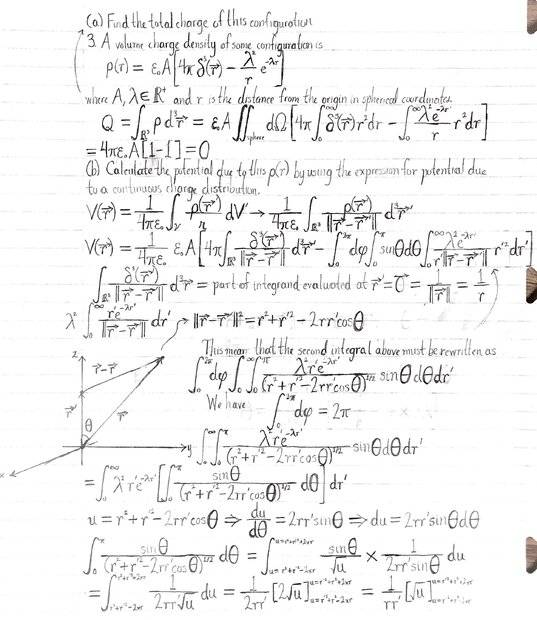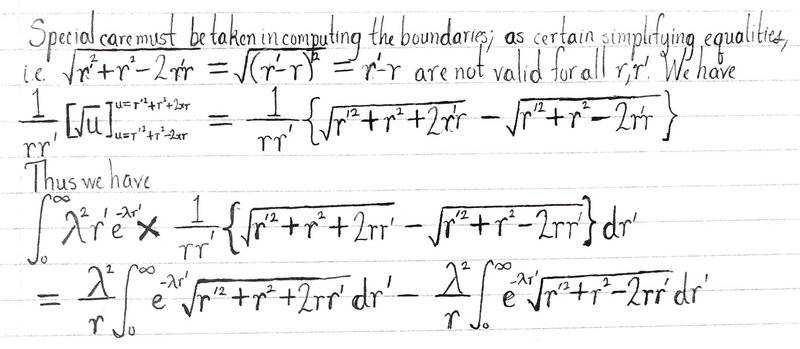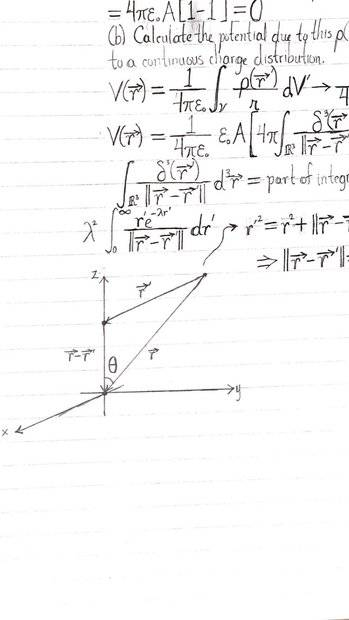# Calculation of Electrostatic Potential Given a Volume Charge Density

• cwill53
In summary, the conversation discusses the difficulties in applying the equations for total charge and electric potential to a given charge distribution. The difficulty arises in evaluating the second integral, which requires splitting into two parts due to the limits of integration. The final part of the conversation discusses finding the divergence of the electric field and how it relates to the original charge density.f

#### cwill53

Homework Statement
This problem is a rehash of Problem 2.50 of the book Introduction to Electrodynamics by Griffiths.

A volume charge density of some configuration is
$$\rho (r)=\epsilon _0A\left [ 4\pi \delta ^3(\mathbf{r})-\frac{\lambda ^2}{r}e^{-\lambda r} \right ]$$

where ##A## and ##\lambda ## are positive constants, and ##r## is the distance from origin in spherical coordinates.
(a) Find the total charge of this configuration.
(b) Calculate the potential due to this ##\rho (r)## by using the expression for the potential
due to a continuous charge distribution.
(c) Calculate the electric field ##E## directly from the potential and verify that the
differential form of Gauss’s law is obeyed.
Relevant Equations
$$Q=\int_{\mathbb{R}^3}^{}\rho \, d^3\mathbf{r}$$

$$V(\mathbf{r})=\frac{1}{4\pi \epsilon _0}\int_{\Gamma }^{}\frac{\rho (\mathbf{r'})}{\left \| \mathbf{r-r'} \right \|}d^3\mathbf{r'}$$

I know for sure that the desired potential is

$$V(\mathbf{r})=A\frac{e^{-\lambda r}}{r}$$
Part (a) was simple, after applying

$$Q=\int_{\mathbb{R}^3}^{}\rho \, d^3\mathbf{r}$$

I found that the total charge of the configuration was zero.

Part (b) is where the difficulties arise for me. I applied

$$V(\mathbf{r})=\frac{1}{4\pi \epsilon _0}\int_{\Gamma }^{}\frac{\rho (\mathbf{r'})}{\left \| \mathbf{r-r'} \right \|}d^3\mathbf{r'}$$

to the given charge distribution. Here's my work.

$$V(\mathbf{r})=\frac{1}{4\pi \epsilon_0}\epsilon _0A\left [ 4\pi \int_{\mathbb{R}^3}^{}\frac{\delta ^3(\mathbf{r})}{\left \| \mathbf{r-r'} \right \|}d^3\mathbf{r}-\int_{0}^{2\pi }d\phi \int_{0}^{\pi }\sin\theta d\theta \int_{0}^{\infty }\frac{\lambda ^2e^{-\lambda r'}}{r'\left \| \mathbf{r-r'} \right \|}r'^2dr' \right ]$$

$$\int_{\mathbb{R}^3}^{}\frac{\delta ^3(\mathbf{r})}{\left \| \mathbf{r-r'} \right \|}d^3\mathbf{r}=\frac{1}{\left \| \mathbf{r} \right \|}=\frac{1}{r}$$

In order to tackle the second integral I tried to use the law of cosines and stipulate that ##\mathbf{r}## is directed along the z-axis. I included the pictures of my work.

$$\int_{0}^{2\pi }d\phi \int_{0}^{\pi }\sin\theta d\theta \int_{0}^{\infty }\frac{\lambda ^2e^{-\lambda r'}}{r'\left \| \mathbf{r-r'} \right \|}r'^2dr'\rightarrow \int_{0}^{2\pi }d\phi\int_{0}^{\infty }\int_{0}^{\pi }\frac{\lambda ^2r'e^{-\lambda r'}}{(r^2+r'^2-2rr'\cos\theta )^{1/2}}\sin\theta d\theta dr'$$

Given that ##\int_{0}^{2\pi }d\phi =2\pi ##

The problem was evaluation of the integral

$$\int_{0}^{\infty }\int_{0}^{\pi }\frac{\lambda ^2r'e^{-\lambda r'}}{(r^2+r'^2-2rr'\cos\theta )^{1/2}}\sin\theta d\theta dr'$$

$$\int_{0}^{\infty }\int_{0}^{\pi }\frac{\lambda ^2r'e^{-\lambda r'}}{(r^2+r'^2-2rr'\cos\theta )^{1/2}}\sin\theta d\theta dr'=\int_{0}^{\infty }\lambda ^2r'e^{-\lambda r}\left [ \int_{0}^{\pi }\frac{\sin\theta }{(r^2+r'^2-2rr'\cos\theta )^{1/2}}d\theta \right ]dr'$$

Using the substitution ##u=r^2+r'^2-2rr'\cos\theta##, I was able to integrate with respect to ##\theta## and arrive at

$$\int_{0}^{\infty }\lambda ^2r'e^{-\lambda r'}\times \frac{1}{rr'}\left \{ \sqrt{r'^2+r^2+2rr'}-\sqrt{r'^2+r^2-2rr'} \right \}dr'$$

$$\frac{\lambda ^2}{r}\left [ \int_{0}^{\infty }e^{-\lambda r'}\sqrt{r'^2+r^2+2rr'}dr'-\int_{0}^{\infty }e^{-\lambda r'}\sqrt{r'^2+r^2-2rr'}dr' \right ]$$

Note that I know for sure that the desired potential is

$$V(\mathbf{r})=A\frac{e^{-\lambda r}}{r}$$

However, the last integrals I arrived at, as far as I know, don't yield the answer I'm looking for. The exponential factor ends up canceling out entirely.You need to be careful with the second integral $$I_2=\int_{0}^{\infty }e^{-\lambda r'}\sqrt{r'^2+r^2-2rr'}dr'$$ Remember that you integrate from zero to infinity, in which case ##r'## can be smaller or greater than ##r##. Then depending on where ##r'## is,
$$+\sqrt{r'^2+r^2-2rr'}=+\sqrt{(r-r')^2}= \begin{cases} -(r-r') & \text{if } r < r' \\ +(r-r') & \text{if } r \geq r' \end{cases}$$ which means that you need to split the integral into two, one with limits from zero to ##r## and one with limits from ##r## to infinity. The first integral does not have this wrinkle because ##+\sqrt{r'^2+r^2+2rr'}=+(r+r').##

•cwill53, Orodruin and topsquark
You need to be careful with the second integral $$I_2=\int_{0}^{\infty }e^{-\lambda r'}\sqrt{r'^2+r^2-2rr'}dr'$$ Remember that you integrate from zero to infinity, in which case ##r'## can be smaller or greater than ##r##. Then depending on where ##r'## is,
$$+\sqrt{r'^2+r^2-2rr'}=+\sqrt{(r-r')^2}= \begin{cases} -(r-r') & \text{if } r < r' \\ +(r-r') & \text{if } r \geq r' \end{cases}$$ which means that you need to split the integral into two, one with limits from zero to ##r## and one with limits from ##r## to infinity. The first integral does not have this wrinkle because ##+\sqrt{r'^2+r^2+2rr'}=+(r+r').##
Thank you so much.

You need to be careful with the second integral $$I_2=\int_{0}^{\infty }e^{-\lambda r'}\sqrt{r'^2+r^2-2rr'}dr'$$ Remember that you integrate from zero to infinity, in which case ##r'## can be smaller or greater than ##r##. Then depending on where ##r'## is,
$$+\sqrt{r'^2+r^2-2rr'}=+\sqrt{(r-r')^2}= \begin{cases} -(r-r') & \text{if } r < r' \\ +(r-r') & \text{if } r \geq r' \end{cases}$$ which means that you need to split the integral into two, one with limits from zero to ##r## and one with limits from ##r## to infinity. The first integral does not have this wrinkle because ##+\sqrt{r'^2+r^2+2rr'}=+(r+r').##
I'm wondering how I should answer part (c) of this question to get something equivalent to the original charge density divided by the vacuum permittivity for the divergence of the electric field.

$$V(\mathbf{r})=A\frac{e^{-\lambda r}}{r}$$

$$\mathbf{E}=-\nabla V=-\hat{\mathbf{r}}\frac{\partial V}{\partial r}(r)=A\frac{e^{-\lambda r}}{r^2}(\lambda r+1)\hat{\mathbf{r}}$$

$$\nabla\cdot \mathbf{E}=\frac{1}{r^2}\frac{\partial }{\partial r}(r^2E_r)=\frac{A}{r^2}\frac{\partial }{\partial r}\left ( \frac{r^2}{r^2}e^{-\lambda r} (\lambda r+1)\right )=-A\frac{\lambda ^2e^{-\lambda r}}{r}$$

But the original charge density is

$$\rho (r)=\epsilon _0A\left [ 4\pi \delta ^3(\mathbf{r})- \frac{\lambda ^2}{r}e^{-\lambda r}\right ]$$

$$\nabla\cdot \mathbf{E}=\frac{1}{r^2}\frac{\partial }{\partial r}(r^2E_r)=\frac{A}{r^2}\frac{\partial }{\partial r}\left ( \frac{r^2}{r^2}e^{-\lambda r} (\lambda r+1)\right )=-A\frac{\lambda ^2e^{-\lambda r}}{r}$$
You are missing the delta function which is zero everywhere except at the origin. Your expression for the divergence is good except at the origin. Is ##\dfrac{r^2}{r^2}=1## at ##r=0##? I think not.

•cwill53
You are missing the delta function which is zero everywhere except at the origin. Your expression for the divergence is good except at the origin. Is ##\dfrac{r^2}{r^2}=1## at ##r=0##? I think not.
Right, right, thanks a ton.